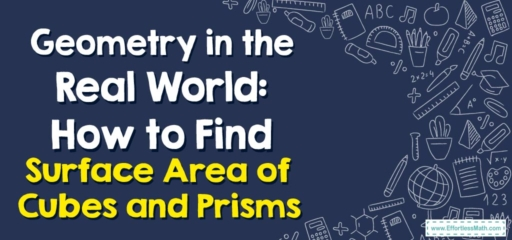# Geometry in the Real World: How to Find Surface Area of Cubes and Prisms

Hello, math enthusiasts!

Today, we're taking a journey into geometry to understand how to calculate the surface area of cubes and prisms. Whether you're wrapping a gift box or painting a wall, understanding surface area is a handy skill to have!## 1. Understanding Surface Area

Surface area refers to the total area that the surface of an object occupies. It’s like the ‘skin’ of the object. For cubes and prisms, it’s calculated by adding up the areas of all the faces.

## 2. Calculating the Surface Area of Cubes and Prisms

Calculating surface area involves adding up the areas of all the faces of the shape.

### Step-By-Step Guide to Calculating the Surface Area of Cubes and Prisms

Let’s break it down:

#### Step 1: Identify the Faces

A cube has six identical square faces, while a rectangular prism has three pairs of identical rectangular faces.

#### Step 2: Calculate the Area of Each Face

For a cube with side length s, each face has an area of $$s^2$$. For a rectangular prism, you’ll calculate the area of each pair of faces ($$length\times width, width\times height, and length\times height$$).

#### Step 3: Sum the Areas of All Faces

This gives you the total surface area of the shape.

For example, let’s calculate the surface area of a cube with a side length of 4 units:

1. Identify the faces: A cube has six identical square faces.
2. Calculate the area of each face: Each face has an area of $$4^2 = 16$$ square units.
3. Sum the areas of all faces: The total surface area is $$16\times 6 = 96$$ square units.

And that’s how you calculate the surface area of cubes and prisms!

As always, keep practicing, keep exploring, and enjoy your mathematical journey!

In this blog post, we’ve explained how to calculate the surface area of cubes and prisms, providing a step-by-step guide to make this mathematical skill easy to understand and implement. With practice, you’ll master this in no time. Happy calculating!

### What people say about "Geometry in the Real World: How to Find Surface Area of Cubes and Prisms - Effortless Math: We Help Students Learn to LOVE Mathematics"?

No one replied yet.

X
30% OFF

Limited time only!

Save Over 30%

SAVE $5 It was$16.99 now it is \$11.99